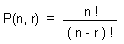Question: What is Permutation ? Answer: A rearrangement of a number of objects is called a permutation. Suppose Ann, Bob and Carole are to sit on three chairs side by side. Then there are 6 different orders in which they could be placed on the chairs, each being a permutation of the 3 people. ABC BCA CAB BAC CBA ACB. There are two main notations for such permutations. In relation notation, one can just arrange the natural ordering of the elements being permuted on a row, and the new ordering on another row. The general case is given by the formula :where, n is the number of elements available for selection, and r is the number of elements to be selected.